Courses

# Rotation about a Fixed Axis and Moving Axis Physics Notes | EduRev

## Physics : Rotation about a Fixed Axis and Moving Axis Physics Notes | EduRev

The document Rotation about a Fixed Axis and Moving Axis Physics Notes | EduRev is a part of the Physics Course Basic Physics for IIT JAM.
All you need of Physics at this link: Physics

Consider a rigid body that is free to rotate about an axis fixed in space. Because of the body’s inertia, it resists being set into rotational motion, and equally important, once rotating, it resists being brought to rest. Exactly how that inertial resistance depends on the mass and geometry of the body is discussed here.
Take the axis of rotation to be the z-axis. A vector in the x-y plane from the axis to a bit of mass fixed in the body makes an angle θ with respect to the x-axis. If the body is rotating, θ changes with time, and the body’s angular frequency is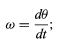(72)

ω is also known as the angular velocity. If ω is changing in time, there is also an angular acceleration α, such that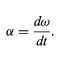(73)

Because linear momentum p is related to linear speed v by p = mv, where m is the mass, and because force F is related to acceleration a by F = ma, it is reasonable to assume that there exists a quantity I that expresses the rotational inertia of the rigid body in analogy to the way m expresses the inertial resistance to changes in linear motion. One would expect to find that the angular momentum is given by
L = Iω    (74)
and that the torque (twisting force) is given by
τ = Iα    (75)

One can imagine dividing the rigid body into bits of mass labeled m1m2m3, and so on. Let the bit of mass at the tip of the vector be called mi, as indicated in Figure 21. If the length of the vector from the axis to this bit of mass is Ri, then mi’s linear velocity vi equals ωR(see equation ) , and its angular momentum Li equals miviRi , (see equation ), or miRi2ω. The angular momentum of the rigid body is found by summing all the contributions from all the bits of mass labeled i = 1, 2, 3 . . . :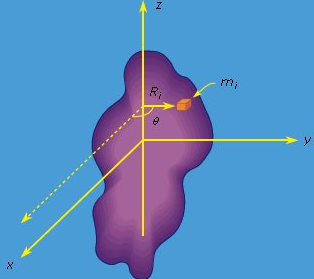Figure 21: Rotation around a fixed axis.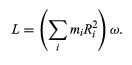(76)

In a rigid body, the quantity in parentheses in equation is always constant (each bit of mass mi always remains the same distance Ri from the axis). Thus if the motion is accelerated, then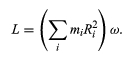(76)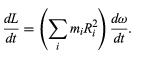(77)

Recalling that τ = dL/dt, one may write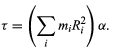(78)

(These equations may be written in scalar form, since L and τ are always directed along the axis of rotation in this discussion.) Comparing equations, (76) and (78) with (74) and (75), one finds that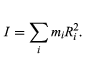(79)

The quantity I is called the moment of inertia.
According to equation, the effect of a bit of mass on the moment of inertia depends on its distance from the axis. Because of the factor Ri2, mass far from the axis makes a bigger contribution than mass close to the axis. It is important to note that Ri is the distance from the axis, not from a point. Thus, if xi and yi are the x and y coordinates of the mass mi, then Ri2 = xi2 + yi2, regardless of the value of the z coordinate. The moments of inertia of some simple uniform bodies are given in the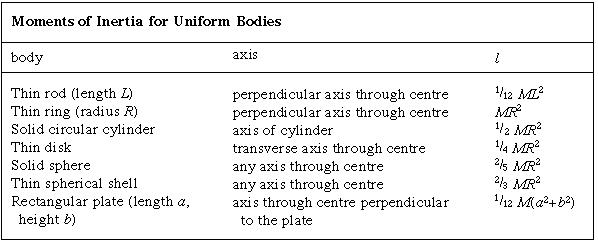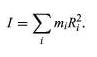(79)

The moment of inertia of any body depends on the axis of rotation. Depending on the symmetry of the body, there may be as many as three different moments of inertia about mutually perpendicular axes passing through the centre of mass. If the axis does not pass through the centre of mass, the moment of inertia may be related to that about a parallel axis that does so. Let Ic be the moment of inertia about the parallel axis through the centre of mass, r the distance between the two axes, and M the total mass of the body. Then
I = Ic + Mr2    (80)

In other words, the moment of inertia about an axis that does not pass through the centre of mass is equal to the moment of inertia for rotation about an axis through the centre of mass (Ic) plus a contribution that acts as if the mass were concentrated at the centre of mass, which then rotates about the axis of rotation.
The dynamics of rigid bodies rotating about fixed axes may be summarized in three equations. The angular momentum is L = , the torque is τ = , and the kinetic energy is K = 1/22.

The general motion of a rigid body tumbling through space may be described as a combination of translation of the body’s centre of mass and rotation about an axis through the centre of mass. The linear momentumof the body of mass M is given by
p = mvc     (81)

where vc is the velocity of the centre of mass. Any change in the momentum is governed by Newton’s second law, which states that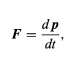(82)

where F is the net force acting on the body. The angular momentum of the body with respect to any reference point may be written as
L = Lc + r × p   (83)

where Lc is the angular momentum of rotation about an axis through the centre of mass, r is a vector from the reference point to the centre of mass, and r × p is therefore the angular momentum associated with motion of the centre of mass, acting as if all the body’s mass were concentrated at that point. The quantity Lc in equation (83) is sometimes called the body’s spin, and r × p is called the orbital angular momentum. Any change in the angular momentum of the body is given by the torque equation,
L = Lc + r × p    (83)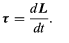(84)

An example of a body that undergoes both translational and rotational motion is the Earth, which rotates about an axis through its centre once per day while executing an orbit around the Sun once per year. Because the Sun exerts no torque on the Earth with respect to its own centre, the orbital angular momentum of the Earth is constant in time. However, the Sun does exert a small torque on the Earth with respect to the planet’s centre, owing to the fact that the Earth is not perfectly spherical. The result is a slow shifting of the Earth’s axis of rotation, known as the precession of the equinoxes (see below).
The kinetic energy of a body that is both translating and rotating is given by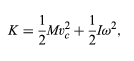(85)

where I is the moment of inertia and ω is the angular velocity of rotation about the axis through the centre of mass.
A common example of combined rotation and translation is rolling motion, as exhibited by a billiard ball rolling on a table, or a ball or cylinder rolling down an inclined plane. Consider the latter example, illustrated in Figure 22. Motion is impelled by the force of gravity, which may be resolved into two components, FN, which is normal to the plane, and Fp, which is parallel to it. In addition to gravity, friction plays an essential role. The force of friction, written as f, acts parallel to the plane, in opposition to the direction of motion, at the point of contact between the plane and the rolling body. If f is very small, the body will slide without rolling. If f is very large, it will prevent motion from occurring. The magnitude of f depends on the smoothness and composition of the body and the plane, and it is proportional to FN, the normal component of the force.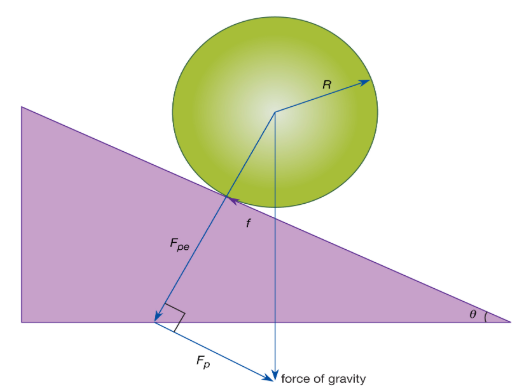Figure 22: Rolling motion.

Consider a case in which f is just large enough to cause the body (sphere or cylinder) to roll without slipping. The motion may be analyzed from the point of view of an axis passing through the point of contact between the rolling body and the plane. Remarkably, the point of contact may always be regarded to be instantaneously at rest. To understand why, suppose that the rolling body has radius R and angular velocity ω about its centre-of-mass axis. Then, with respect to its own axis, each point on the circular cross section in Figure 22 moves with instantaneous tangential linear speed vc = . In particular, the point of contact is moving backward with this speed relative to the centre of mass. But with respect to the inclined plane, the centre of mass is moving forward with exactly this same speed. The net effect of the two equal and opposite speeds is that the point of contact is always instantaneously at rest. Therefore, although friction acts at that point, no work is done by friction, so mechanical energy (potential plus kinetic) may be regarded as conserved.
With respect to the axis through the point of contact, the torque is equal to RFp, giving rise to an angular acceleration α given by Ipα = RFp, where Ip is the moment of inertia about the point-of-contact axis and can be determined by applying equation 80 relating moments of inertia about parallel axes (Ip = I + MR2). Thus,
I = Ic + Mr2   (80)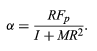(86)

From this result, the motion of the body is easily obtained using the fact that the velocity of the centre of mass is vc = and hence the linear acceleration of the centre of mass is ac = .
Notice that, although without friction no angular acceleration would occur, the force of friction does not affect the magnitude of α. Because friction does no work, this same result may be obtained by applying energy conservation. The situation also may be analyzed entirely from the point of view of the centre of mass. In that case, the torque is −fR, but f also provides a linear force on the body. The f may then be eliminated by using Newton’s second law and the fact that the torque equals the moment of inertia times the angular acceleration, once again leading to the same result.
One more interesting fact is hidden in the form of equation (86). The parallel component of the force of gravity is given by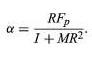(86)

Fp = Mg sinθ     (87)
where θ is the angle of inclination of the plane. The moment of inertia about the centre of mass of any body of mass M may be written
I = Mk2            (88)

where k is a distance called the radius of gyration. Comparison to equation (79) shows that k is a measure of how far from the centre of mass the mass of the body is concentrated. Using equations (87) and (88) in equation (86), one finds that
Fp = Mg sinθ   (87)
I = Mk2            (88)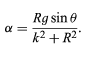(89)

Thus, the angular acceleration of a body rolling down a plane does not depend on its total mass, although it does depend on its shape and distribution of mass. The same may be said of ac, the linear acceleration of the centre of mass. The acceleration of a rolling ball, like the acceleration of a freely falling object, is independent of its mass. This observation helps to explain why Galileo was able to discover many of the basic laws of dynamicsin gravity by studying the behaviour of balls rolling down inclined planes.

Offer running on EduRev: Apply code STAYHOME200 to get INR 200 off on our premium plan EduRev Infinity!

,

,

,

,

,

,

,

,

,

,

,

,

,

,

,

,

,

,

,

,

,

;